# E-learning in analysis of genomic and proteomic data 2. Data analysis2.2. Analysis of high-density genomic data2.2.1. DNA microarrays2.2.1.8. Analysis of arrayCGH

Some other authors believe that smoothing techniques are sufficient for searching for changed regions (; ; ; ).

In general, the process of smoothing is similar to fitting the model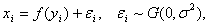where xi denotes the observed value and yi the position of the clone.

A wide variety of smoothers has been proposed so far. Again, they differ in the distribution assumptions, objective functions and optimization algorithms to fit the model. One of the first suitable approaches was the quantile smoother proposed in . This type of smoother dates back to  and was used by Schimek  as graphical technique for the first time. The main difference to other smoothers is a non-standard definition of its objective function (e.g. applying the sum of absolute values instead of the sum of squares). Thus features in the curve, for instance sudden jumps or flat plateaus can be emphasized to appear more distinct, which is an advantage when processing arrayCGH data. In quantile smoothing the objective function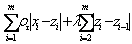is minimized, where the xi‘s are the raw log2ratio values, the zi‘s are the fitted values,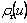is the so-called check-function such thatwhen u>0 and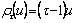when u<=0, and t a quantile of interest. l is a tuning parameter that controls the bias-variance tradeoff (increased l values produce smoother fits). Apart from l = 0.5 (i.e. the median), Eilers & de Menezes  considered also l = 0.15 and l = 0.85 for the detection of wide chromosomal changes. The idea is to obtain bounds that allow the identification of small local changes that are expected to lie outside of the bounded area. Both types of alterations are detected if they exceed pre-specified threshold values as suggested in .

Another promising approach allowing for abrupt function changes is wavelet smoothing as proposed in . They suggested to fit wavelets to the data and thus to shrink the coefficients. This means that in the flat parts most of the higher frequency coefficients will become zero, but near the jumps they will be retained. The breakpoints are estimated in that way. After the breakpoints are estimated, the data in thus obtained segments are averaged concluding in the final result. Huang et al.  proposed a robust quantile smoothing procedure based on a double heavy-tailed random effect model. Li & Zhu  established the concept of fused quantile regression which takes additionally into account the real distances of the clones on the genome by using divided differences,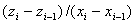in the penalty of the objective function. Moreover, they proposed a method for the selection of the smoothing parameter l in this situation. As for the real distance of clones, Eilers & de Menezes  pointed out that if one minimizes the sum of absolute values in the optimization function, the derivative is the sum of signs and thus only the signs matter. Because clones are ordered such thatcorrecting for distances between adjacent clones will not change the signs and thus will give the same results as in the non-corrected case.

Even when smoothing, especially in rather noisy data, is capable of enhancing the signal, we can never expect more than a de-noised data set. Hence it is not surprising that according to the comparative study of Lai et al. , smoothing techniques have proven to provide better detection results for highly noisy data and quite small aberrated regions than other types of methods. Smoothing methods are designed rather for graphical inspection of the data than for automated identification of aberrated regions. Eilers & de Menezes  suggest letting the user specify a cutoff to detect segmented regions following quantile smoothing, however, this includes the risk of an unintentional non-inclusion of some altered clones as already discussed in this paper. On the other hand, according to , segmentation methods appear to perform consistently well, and, what is also important, are more straightforward to interpret. Lai et al.  suggest that the optimal combination of a smoothing step and a segmentation step might improve the overall performance. We are convinced that for a suitable method it is necessary to automatically detect aberrated regions, even when the data are very noisy.